## Tools for Graphing Functions, Curves, and Surfaces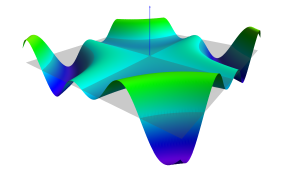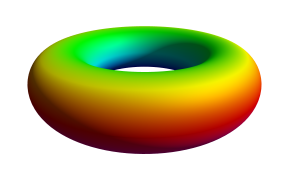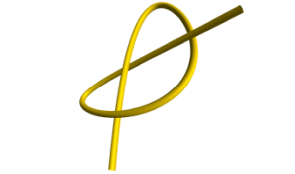function from $\mathbb{R}^2$ to $\mathbb{R}$ two-dimensional surface in $\mathbb{R}^3$ one-dimensional curve in $\mathbb{R}^3$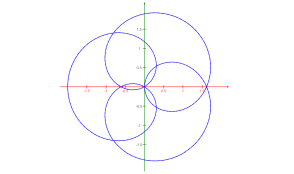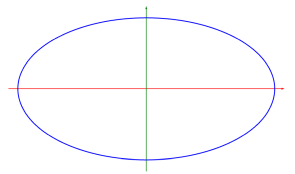function from $\mathbb{R}$ to $\mathbb{R}$ one-dimensional curve in $\mathbb{R}^2$ implicit curve in $\mathbb{R}^2$

The links above offer various ways to graph functions, curves, and surfaces. They were inspired by Lee Stemkoski's example code and they use MathBox by Steve Wittens as well as three.js, dat.GUI, and Matthew Crumley's JavaScript Expression Evaluator. They will currently work best on desktop computers using Chrome. Firefox should also be OK, but might sometimes be slow.

I think most of this should be rather self-explanatory, but here are some hints:

• Functions can be entered in two ways. One is a kind of "abbreviated JavaScript" where you can, for example, write sin(x) instead of Math.sin(x) or 2^x instead of Math.pow(2,x). (For more details see here.) The other way is to write proper JavaScript. This should only be necessary for pretty complicated functions which need temporary variables, loops, or conditional statements. (See example below.)
So, for the function $x \mapsto \cos x^3$ you either write cos(x^3) or return Math.cos(Math.pow(x,3)). The code decides how to parse your input by looking for the keyword return.
• The code uses three.js OrbitControls so that you can use your mouse to move the "camera". Move the mouse with the left button pressed to change the viewing angle. Move the mouse with the right button pressed for panning. Use the scroll wheel for zooming.
• The axes are colored red, green, and blue for $\color{red}x$, $\color{green}y$, and $\color{blue}z$; mnemonic: RGB.
• Note that axes will only be shown if the signs of the corresponding min/max values differ. For example, if xmin and xmax are both positive, then neither the $y$ axis nor the $z$ axis are shown.
• The code doesn't do a lot of error checking. If, say, your xmin value is greater than your xmax value, it won't warn you, but you probably won't see anything interesting.
• dat.GUI sometimes makes it seem as if you can't enter digits right of the decimal point. But you can enter as many as you like: you'll just have to finish the input with the enter key.
• If you want to be able to reproduce a specific graph with all settings, open a console in your web browser and call the function createUrl with no arguments. It should return a URL for what you currently see on your screen.

Here are some examples (play with the parameters $a$ and $b$ if possible):

Copyright (c) 2016-2017, Prof. Dr. Edmund WeitzImpressum, Datenschutzerklärung.